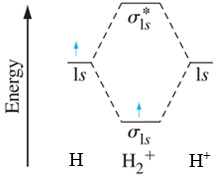# Homework #8

$$\newcommand{\vecs}{\overset { \rightharpoonup} {\mathbf{#1}} }$$ $$\newcommand{\vecd}{\overset{-\!-\!\rightharpoonup}{\vphantom{a}\smash {#1}}}$$$$\newcommand{\id}{\mathrm{id}}$$ $$\newcommand{\Span}{\mathrm{span}}$$ $$\newcommand{\kernel}{\mathrm{null}\,}$$ $$\newcommand{\range}{\mathrm{range}\,}$$ $$\newcommand{\RealPart}{\mathrm{Re}}$$ $$\newcommand{\ImaginaryPart}{\mathrm{Im}}$$ $$\newcommand{\Argument}{\mathrm{Arg}}$$ $$\newcommand{\norm}{\| #1 \|}$$ $$\newcommand{\inner}{\langle #1, #2 \rangle}$$ $$\newcommand{\Span}{\mathrm{span}}$$ $$\newcommand{\id}{\mathrm{id}}$$ $$\newcommand{\Span}{\mathrm{span}}$$ $$\newcommand{\kernel}{\mathrm{null}\,}$$ $$\newcommand{\range}{\mathrm{range}\,}$$ $$\newcommand{\RealPart}{\mathrm{Re}}$$ $$\newcommand{\ImaginaryPart}{\mathrm{Im}}$$ $$\newcommand{\Argument}{\mathrm{Arg}}$$ $$\newcommand{\norm}{\| #1 \|}$$ $$\newcommand{\inner}{\langle #1, #2 \rangle}$$ $$\newcommand{\Span}{\mathrm{span}}$$

## Q1

Draw all the resonance Lewis structures of $$ClO_3^-$$ and identify the bond order of the chlorine/oxygen bonds.

## Q2

Complate the following table. For the Lewis structures, minimize formal charges whenever possible (make formal charges as close to zero as possible). For electron group geometry and molecular geometry, answer with respect to the UNDERLINED ATOM ONLY. When multiple resonance Lewis structures are present, draw only one structure. Note: “Cl” in the below molecules is chlorine, not C (Carbon) and I (iodine).

 Species Lewis structure # of electron groups # of atoms bonded to central atom electron group geometry molecular geometry bond angles Polar or non-polar $$H_2\underline{O}$$ $$\underline{Cl}O_4^{-}$$ $$\underline{I}Cl_4^+$$ $$\underline{Xe}F_4$$ $$\underline{C}H_2CH_2$$

## Q3

As an example, the molecular orbital (MO) energy diagram for $$H_2^+$$ is shown below.In a similar fashion, draw the molecular orbital energy diagram for $$O_2^+$$.

1. Fill in the electrons using arrows (↑ and/or ↓) for the atomic and molecular orbitals in the MO diagram.
2. Designate all the energy levels (i.e., $$\sigma_{2s}$$,$$\pi_{2p}$$, etc) in the MO diagram.
3. Calculate the bond order for $$O_2^+$$.
4. Is $$O_2^+$$ a paramagnetic or diamagnetic chemical species?

Homework #8 is shared under a not declared license and was authored, remixed, and/or curated by LibreTexts.